xParabolic trajectoryEncyclopedia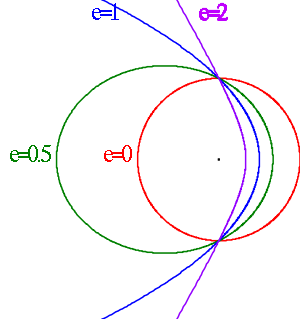In astrodynamics
Astrodynamics
Orbital mechanics or astrodynamics is the application of ballistics and celestial mechanics to the practical problems concerning the motion of rockets and other spacecraft. The motion of these objects is usually calculated from Newton's laws of motion and Newton's law of universal gravitation. It...

or celestial mechanics
Celestial mechanics
Celestial mechanics is the branch of astronomy that deals with the motions of celestial objects. The field applies principles of physics, historically classical mechanics, to astronomical objects such as stars and planets to produce ephemeris data. Orbital mechanics is a subfield which focuses on...

a parabolic trajectory is a Kepler orbit
Kepler orbit
In celestial mechanics, a Kepler orbit describes the motion of an orbiting body as an ellipse, parabola, or hyperbola, which forms a two-dimensional orbital plane in three-dimensional space...

with the eccentricity
Orbital eccentricity
The orbital eccentricity of an astronomical body is the amount by which its orbit deviates from a perfect circle, where 0 is perfectly circular, and 1.0 is a parabola, and no longer a closed orbit...

equal to 1. When moving away from the source it is called an escape orbit, otherwise a capture orbit. It is also sometimes referred to as a C3 = 0 orbit.

Under standard assumptions a body traveling along an escape orbit will coast along a parabolic
Parabola
In mathematics, the parabola is a conic section, the intersection of a right circular conical surface and a plane parallel to a generating straight line of that surface...

shaped trajectory to infinity, with velocity relative to the central body
Central body
In astrodynamics a central body is a body that is being orbited by an secondary body, or satellite .The central body is properly referred to as the primary body.Under standard assumptions in astrodynamics:...

tending to zero, and therefore will never return. Parabolic trajectory are minimum-energy escape trajectories, separating positive-energy
Characteristic energy
In astrodynamics a characteristic energy , a form of specific energy, is a measure of the energy required for an interplanetary mission that requires attaining an excess orbital velocity over an escape velocity required for additional orbital maneuvers...

hyperbolic trajectories
Hyperbolic trajectory
In astrodynamics or celestial mechanics a hyperbolic trajectory is a Kepler orbit with the eccentricity greater than 1. Under standard assumptions a body traveling along this trajectory will coast to infinity, arriving there with hyperbolic excess velocity relative to the central body. Similarly to...

from negative-energy elliptic orbit
Elliptic orbit
In astrodynamics or celestial mechanics an elliptic orbit is a Kepler orbit with the eccentricity less than 1; this includes the special case of a circular orbit, with eccentricity equal to zero. In a stricter sense, it is a Kepler orbit with the eccentricity greater than 0 and less than 1 . In a...

s.

## Velocity

Under standard assumptions the orbital velocity
Orbital velocity
Orbital velocity can refer to the following:* The orbital speed of a body in a gravitational field.* The velocity of particles due to wave motion, in particular in wind waves....

() of a body travelling along parabolic trajectory can be computed as: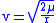where:
•is the radial distance of orbiting body from central body
Central body
In astrodynamics a central body is a body that is being orbited by an secondary body, or satellite .The central body is properly referred to as the primary body.Under standard assumptions in astrodynamics:...

,
•is the standard gravitational parameter
Standard gravitational parameter
In astrodynamics, the standard gravitational parameter μ of a celestial body is the product of the gravitational constant G and the mass M of the body.\mu=GM \ The SI units of the standard gravitational parameter are m3s−2....

.

At any position the orbiting body has the escape velocity
Escape velocity
In physics, escape velocity is the speed at which the kinetic energy plus the gravitational potential energy of an object is zero gravitational potential energy is negative since gravity is an attractive force and the potential is defined to be zero at infinity...

for that position.

If the body has the escape velocity with respect to the Earth, this is not enough to escape the Solar System, so near the Earth the orbit resembles a parabola, but further away it bends into an elliptical orbit around the Sun.

This velocity () is closely related to the orbital velocity
Orbital velocity
Orbital velocity can refer to the following:* The orbital speed of a body in a gravitational field.* The velocity of particles due to wave motion, in particular in wind waves....

of a body in a circular orbit
Circular orbit
A circular orbit is the orbit at a fixed distance around any point by an object rotating around a fixed axis.Below we consider a circular orbit in astrodynamics or celestial mechanics under standard assumptions...

of the radius equal to the radial position of orbiting body on the parabolic trajectory:where:
•is orbital velocity
Orbital velocity
Orbital velocity can refer to the following:* The orbital speed of a body in a gravitational field.* The velocity of particles due to wave motion, in particular in wind waves....

of a body in circular orbit
Circular orbit
A circular orbit is the orbit at a fixed distance around any point by an object rotating around a fixed axis.Below we consider a circular orbit in astrodynamics or celestial mechanics under standard assumptions...

.

## Equation of motion

Under standard assumptions, for a body moving along this kind of trajectory
Orbit
In physics, an orbit is the gravitationally curved path of an object around a point in space, for example the orbit of a planet around the center of a star system, such as the Solar System...

an orbital equation becomes: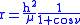where:
•is radial distance of orbiting body from central body
Central body
In astrodynamics a central body is a body that is being orbited by an secondary body, or satellite .The central body is properly referred to as the primary body.Under standard assumptions in astrodynamics:...

,
•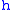is specific angular momentum of the orbiting body
Orbiting body
In astrodynamics, an orbiting body is a body that orbits a primary body .The orbiting body is properly referred to as the secondary body.It is less massive than the primary body ....

,
•is a true anomaly
True anomaly
In celestial mechanics, the true anomaly is an angular parameter that defines the position of a body moving along a Keplerian orbit. It is the angle between the direction of periapsis and the current position of the body, as seen from the main focus of the ellipse .The true anomaly is usually...

of the orbiting body,
•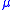is the standard gravitational parameter
Standard gravitational parameter
In astrodynamics, the standard gravitational parameter μ of a celestial body is the product of the gravitational constant G and the mass M of the body.\mu=GM \ The SI units of the standard gravitational parameter are m3s−2....

.

## Energy

Under standard assumptions, specific orbital energy
Specific orbital energy
In the gravitational two-body problem, the specific orbital energy \epsilon\,\! of two orbiting bodies is the constant sum of their mutual potential energy and their total kinetic energy , divided by the reduced mass...

() of parabolic trajectory is zero, so the orbital energy conservation equation for this trajectory takes form: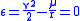where:
•is orbital velocity
Orbital velocity
Orbital velocity can refer to the following:* The orbital speed of a body in a gravitational field.* The velocity of particles due to wave motion, in particular in wind waves....

of orbiting body,
•is radial distance of orbiting body from central body
Central body
In astrodynamics a central body is a body that is being orbited by an secondary body, or satellite .The central body is properly referred to as the primary body.Under standard assumptions in astrodynamics:...

,
•is the standard gravitational parameter
Standard gravitational parameter
In astrodynamics, the standard gravitational parameter μ of a celestial body is the product of the gravitational constant G and the mass M of the body.\mu=GM \ The SI units of the standard gravitational parameter are m3s−2....

.

This is entirely equivalent to the characteristic energy
Characteristic energy
In astrodynamics a characteristic energy , a form of specific energy, is a measure of the energy required for an interplanetary mission that requires attaining an excess orbital velocity over an escape velocity required for additional orbital maneuvers...

(square of the speed at infinity) being 0:A radial parabolic trajectory is a non-periodic trajectory on a straight line
In astrodynamics and celestial mechanics a radial trajectory is a Kepler orbit with zero angular momentum. Two objects in a radial trajectory move directly towards or away from each other in a straight line.- Classification :...

where the relative velocity of the two objects is always the escape velocity
Escape velocity
In physics, escape velocity is the speed at which the kinetic energy plus the gravitational potential energy of an object is zero gravitational potential energy is negative since gravity is an attractive force and the potential is defined to be zero at infinity...

. There are two cases: the bodies move away from each other or towards each other.

There is a rather simple expression for the position as function of time: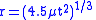where
• μ is the standard gravitational parameter
Standard gravitational parameter
In astrodynamics, the standard gravitational parameter μ of a celestial body is the product of the gravitational constant G and the mass M of the body.\mu=GM \ The SI units of the standard gravitational parameter are m3s−2....

•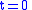corresponds to the extrapolated time of the fictitious starting or ending at the center of the central body.

At any time the average speed fromis 1.5 times the current speed, i.e. 1.5 times the local escape velocity.

To haveat the surface, apply a time shift; for the Earth (and any other spherically symmetric body with the same average density) as central body this time shift is 6 minutes and 20 seconds; seven of these periods later the height above the surface is three times the radius, etc.